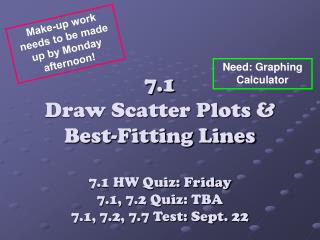DownloadDownload Presentation7.1 Draw Scatter Plots & Best-Fitting Lines 7.1 HW Quiz: Friday 7.1, 7.2 Quiz: TBA 7.1, 7.2, 7.7 Test: Sept. 22

# 7.1 Draw Scatter Plots & Best-Fitting Lines 7.1 HW Quiz: Friday 7.1, 7.2 Quiz: TBA 7.1, 7.2, 7.7 Test: Sept. 22

Télécharger la présentation## 7.1 Draw Scatter Plots & Best-Fitting Lines 7.1 HW Quiz: Friday 7.1, 7.2 Quiz: TBA 7.1, 7.2, 7.7 Test: Sept. 22

- - - - - - - - - - - - - - - - - - - - - - - - - - - E N D - - - - - - - - - - - - - - - - - - - - - - - - - - -
##### Presentation Transcript

1. Make-up work needs to be made up by Monday afternoon! Need: Graphing Calculator 7.1 Draw Scatter Plots & Best-Fitting Lines7.1 HW Quiz: Friday7.1, 7.2 Quiz: TBA7.1, 7.2, 7.7 Test: Sept. 22

2. Vocabulary • Scatter plot: a graph of a set of data pairs (x, y) • Positive correlation:y tends to increase as x increases • Negative correlation:y tends to decrease as x increases

3. More Vocabulary • Correlation coefficient: denoted by r, is a number from -1 to 1 that measures how well a line fits a set of data pairs (x, y). • If r is near 1, the points lie close to a line with a positive slope. • If r is near -1, the points lie close to a line with a negative slope. • If r is near 0, the points do not lie close to any line.

4. y y y y y x x x x x Correlation Coefficient: • What do you think the correlation coefficient would be for these graphs?

5. If “r” is… • -.956 • .899 • 1 • 0 • -1 • 0.5 • -0.6

6. Guided Practice for Ex.1, p. 248 For each scatter plot, (a) tell whether the datahave a positive, negative, or approximately no correlation, and (b) tell whether the correlation is closest to -1, -0.5, 0, 0.5, or 1. 1) 2)

7. More Vocabulary • Best-fitting line: line that lies as close as possible to all the data points • Linear regression: a method for finding the equation of the best-fitting line, or regression, which expresses the linear relationship between the independent variable x and the dependent variable, y, e.g. y = ax + b

8. Example 2: Approximate the best-fitting line • Scatterplot • Best-Fit Line EQ • Best-Fit Line Graph See Handout

9. Guided Practice for Example 2: p. 249 • Draw a scatter plot and approximate the best-fitting line for the following data: (1, 22), (2, 30), (3, 33), (4, 39), (5, 45) y = 5.5x + 17.3

10. Start Homework NOW! • P. 250-251 #1-2, 7-9 (may use graphing calculator) • Finish PSAT Practice WS will turn in tomorrow! (show work!)